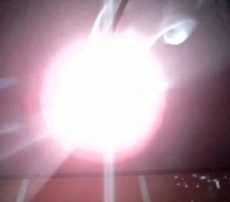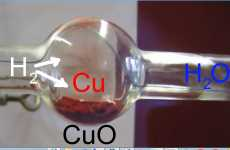Search:

# Oxidations and reductions

## Oxidation of nonmetals by $O_2$

### The reaction of carbon with oxygen:Carbon burns with a yellow flame and sparks producing carbon dioxide, a colourless and odourless gas. $C$ $+$ $O_2$ $\longrightarrow$ $CO_2$

### The reaction of white phosphorous with oxygen:White phosphorous burns with very intense yellow flame producing a thick white smoke of phosporous(V) oxide. $P_4$ $+$ $5O_2$ $\longrightarrow$ $P_4O_{10}$

### The rection of sulfur with oxygen:Sulfur burns with a blue flame producing sulfur dioxide ( = sulfur(IV)oxide ), a colourless gas with a suffocationg odour. $S_8$ $+$ $8O_2$ $\longrightarrow$ $8SO_2$

## Oxidation of metals by $O_2$

### The reaction of magnesium with oxygen:Magnesium burns with a dazzling white flame producing magnesium oxide, a white solid substance. $2Mg$ $+$ $O_2$ $\longrightarrow$ $2MgO$

### The reaction of sodium with oxygen:Sodium burns with a yellow flame producing sodium oxide, a white solid substance. $4Na$ $+$ $O_2$ $\longrightarrow$ $2Na_2O$

## Reactions of oxides with water

### Oxides of nonmetals

The oxides of nonmetals will cause the aqueous solution of bromothymol to change to yellow. Acids are produced: $CO_2$ $+$ $H_2O$ $\longrightarrow$ $H_2CO_3$ (product: carbonic acid) $P_4O_{10}$ $+$ $6H_2O$ $\longrightarrow$ $4H_3PO_4$ (product: phosphoric acid) $SO_2$ $+$ $H_2O$ $\longrightarrow$ $H_2SO_3$ (product: sulfurous acid)

### Oxides of metals

The oxides of metals will cause the aqueous solution of bromothymol to change to blue. Bases are produced: $MgO$ $+$ $H_2O$ $\longrightarrow$ $Mg(OH)_2$ (product: magnesium hydroxyde) $Na_2O$ $+$ $H_2O$ $\longrightarrow$ $2NaOH$ (product: sodium hydroxyde)

## Reduction of metal oxides by $H_2$

### The reaction of copper(II) oxide with hydrogenHeated in a stream of hydrogen, the black powder of copper(II) oxide turns to red copper powder. Water has been produced which condenses on the cold tube wall. $CuO$ $+$ $H_2$ $\longrightarrow$ $Cu$ $+$ $H_2O$

### The reaction of iron(III) oxide with hydrogenHeated in a stream of hydrogen, the red powder of iron(III) oxide (= rust) turns to black iron powder. Water has been produced which condenses on the cold tube wall. $Fe_2O_3$ $+$ $3H_2$ $\longrightarrow$ $2Fe$ $+$ $3H_2O$

## Oxidation and reduction

During oxidation oxygen atoms are added to the substance which is oxidised to form oxides. During reduction, oxygen atoms are removed from the substance which is reduced.

Oxidation and reduction are opposite processes. Oxygen is one example of an oxidant Hydrogen is one example of a reductant

An oxidant is a substance which oxydises another substance. A reductant is a substance which reduces another substance.